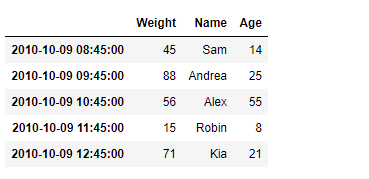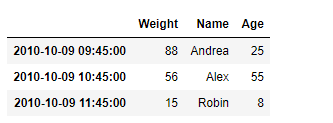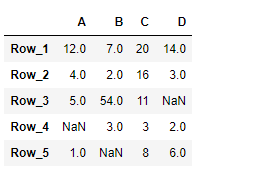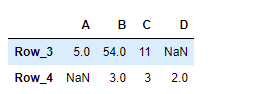GeeksforGeeks App
Open AppBrowser
Continue

# Python | Pandas DataFrame.truncate

Pandas DataFrame is a two-dimensional size-mutable, potentially heterogeneous tabular data structure with labeled axes (rows and columns). Arithmetic operations align on both row and column labels. It can be thought of as a dict-like container for Series objects. This is the primary data structure of the Pandas.

Pandas` DataFrame.truncate()` function is used to truncate a Series or DataFrame before and after some index value. This is a useful shorthand for boolean indexing based on index values above or below certain thresholds.

Syntax: DataFrame.truncate(before=None, after=None, axis=None, copy=True)

Parameter :
before : Truncate all rows before this index value.
after : Truncate all rows after this index value.
axis : Axis to truncate. Truncates the index (rows) by default.
copy : Return a copy of the truncated section.

Returns : The truncated Series or DataFrame.

Example #1: Use `DataFrame.truncate()` function to truncate some entries before and after the passed labels of the given dataframe.

 `# importing pandas as pd``import` `pandas as pd`` ` `# Creating the DataFrame``df ``=` `pd.DataFrame({``'Weight'``:[``45``, ``88``, ``56``, ``15``, ``71``],``                   ``'Name'``:[``'Sam'``, ``'Andrea'``, ``'Alex'``, ``'Robin'``, ``'Kia'``],``                   ``'Age'``:[``14``, ``25``, ``55``, ``8``, ``21``]})`` ` `# Create the index``index_ ``=` `pd.date_range(``'2010-10-09 08:45'``, periods ``=` `5``, freq ``=``'H'``)`` ` `# Set the index``df.index ``=` `index_`` ` `# Print the DataFrame``print``(df)`

Output :Now we will use `DataFrame.truncate()` function to truncate the entries before ‘2010-10-09 09:45:00’ and after ‘2010-10-09 11:45:00’ in the given dataframe.

 `# return the truncated dataframe``result ``=` `df.truncate(before ``=` `'2010-10-09 09:45:00'``, after ``=` `'2010-10-09 11:45:00'``)`` ` `# Print the result``print``(result)`

Output :As we can see in the output, the `DataFrame.truncate()` function has successfully truncated the entries before and after the passed labels in the given dataframe.

Example #2: Use `DataFrame.truncate()` function to truncate some entries before and after the passed labels of the given dataframe.

 `# importing pandas as pd``import` `pandas as pd`` ` `# Creating the DataFrame``df ``=` `pd.DataFrame({``"A"``:[``12``, ``4``, ``5``, ``None``, ``1``], ``                   ``"B"``:[``7``, ``2``, ``54``, ``3``, ``None``], ``                   ``"C"``:[``20``, ``16``, ``11``, ``3``, ``8``], ``                   ``"D"``:[``14``, ``3``, ``None``, ``2``, ``6``]}) `` ` `# Create the index``index_ ``=` `[``'Row_1'``, ``'Row_2'``, ``'Row_3'``, ``'Row_4'``, ``'Row_5'``]`` ` `# Set the index``df.index ``=` `index_`` ` `# Print the DataFrame``print``(df)`

Output :Now we will use `DataFrame.truncate()` function to truncate the entries before ‘Row_3’ and after ‘Row_4’ in the given dataframe.

 `# return the truncated dataframe``result ``=` `df.truncate(before ``=` `'Row_3'``, after ``=` `'Row_4'``)`` ` `# Print the result``print``(result)`

Output :As we can see in the output, the `DataFrame.truncate()` function has successfully truncated the entries before and after the passed labels in the given dataframe.

My Personal Notes arrow_drop_up